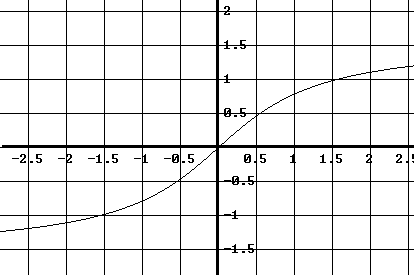# Calculus Questions with Answers (3)

Approximate graphically the first derivative of a function f from its graph. Questions are presented along with solutions.

### Question 1

Below is shown the graph of function f.
a) Assuming that the only extrema of function f are the ones shown in the graph, for what values of x is f '(x) = 0?
b) Assuming that the graph of function f rises indefinitely on the right and on the left, for what values of x is f '(x) negative? For what values of x is f '(x) positive?Solution to Question 1:

• a) The graph of f has two minimums, one at x = -2 and one at x = 4, and one maximum at x = 1. Therefore f '(x) = 0 for x = -2, x = 1 and x = 4.
• b) Function f is decreasing on the intervals (- ∞ , -2) and (1 , 4). Hence f' (x) will be negative on these same intervals.
• Function f is increasing on the intervals (-2 , 1) and (4 , + ∞). Hence f' (x) will be positive on these same intervals.

### Question 2

The graph of function f is shown below. Assuming that function f is odd and has horizontal asymptotes, approximate graphically the graph of the first derivative f ' of f.Solution to Question 2:

• We first note that f is an increasing function. Hence f'(x) is positive for all values of and its graph is above the x axis. The value of the first derivative f '(a) at a given value of x = a is equal to the slope of the tangent line at the point (a , f(a)) . Hence, a possible approximation of f '(x) would be an approximation of the slope of the tangent line at the graph of f. For the given graph, it seems that the slope of the tangent line to the graph of f will be maximum around the origin (0,0).• Let us use three points A, B and C, close to the origin 0 to approximate the slope mo of the tangent line at x = 0. If points A and C have the coordinates A(xA,yA) and C(xC,yC), then mo may be approximated by
mo = (yC - yA) / (xC - xA) = (0.5 - (-0.5))/ (0.5 - (-0.5)) = 1
• We can also use points C and E to approximate the slope m1 of the tangent line at point D.
m1 = (1 - 0.5) / (1.5 - 0.5) = 0.5
• Since f has horizontal asymptotes, we would expect f'(x) to be close to zero as x increases indefinitely (+ ∞) or decreases indefinitely (- ∞).
• Since f has horizontal asymptotes, we would expect the tangent line to the graph of to be horizontal and its slope close to zero as x increases indefinitely (+ ∞) or decreases indefinitely (- ∞).
• Using all the above information, one possible approximation of f'(x) would be as shown below in blue.### Question 3

Approximate the graph of the first derivative f ' of function f when given the graph of f below. Assume that the graph of f is symmetric with respect to the vertical line x = -0.5 and that f has y = 0 as a horizontal asymptote.Solution to Question 3:

• Using similar ideas to those used in questions 1 and 2 above, note that f'(x) = 0 at x = -2, -0.5 and 1 since these are the locations of the extrema of f unction f. The groups of three (green) points are used to approximate the slope of the tangent line at the middle point of each group. For example using points D, E and F one can approximate the slope mo of the tangent line at point E as follows
mo = (0.8 - 0.4) / (-2.4 - (-2.9)) = 0.8
• We can use the next group of the three (green) points, to the right of point A, to approximate the slope at the middle point which will be close to -0.8. The above information may easily be used to plot points on the graph of f' as shown below (blue points).• The sign of f'(x) is determined by the increase and decrease of f. Hence f'(x) is positive for values of on the intervals of increase of f given by:
(- ∞ , -2) and (-0.5 , 1)
• f'(x) is negative for values of x in the intervals of decrease of f and are defined by:
(-2 , -0.5) and (1 , + ∞)
• Using all the above information, we may approximate f'(x) as follows (blue graph).| 1 | 2 | 3 | 4 | 5 |

More references on calculus
questions with answers, tutorials and problems .